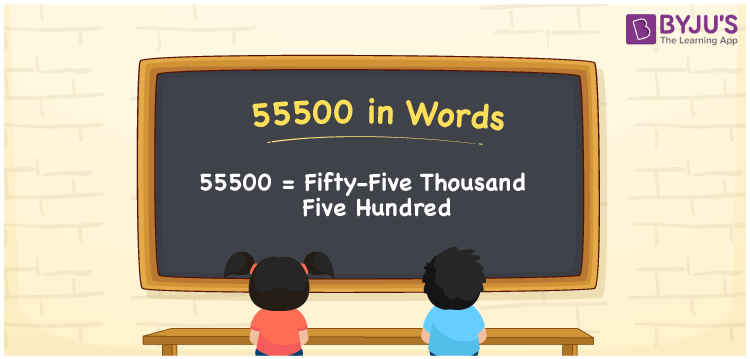# 55500 in Words

55500 in words is Fifty-five Thousand Five Hundred. The number 55500 is a cardinal number and it is also a natural number. For example, the cost of the laptop is Rs. 555000. For converting 555000 into words, we will use the place value system. Go through the below article to learn the spelling of 55500 in words in detail.

 55500 in Words: Fifty-five Thousand Five hundred. Fifty-five Thousand Five hundred in Numerical Form: 55500.

## 55500 in English Words## How to Write 55500 in Words?

The number 55500 place value table is shown below.

 Ten-thousands Thousands Hundreds Tens Ones 5 5 5 0 0

The expanded form of 55500 is as follows:

= 5 × Ten thousands + 5 × Thousand + 5 × Hundred + 0 × Ten + 0 × One

= 5 × 10000 + 5 × 1000 + 5 × 100 + 0 × 10 + 0 × 1

= 50000 + 5000 + 500

= 55500

= Fifty-five thousand five hundred

Hence, 55500 in words is fifty-five thousand five hundred.

55500 in words – Fifty-five thousand five hundred

Is 55500 an odd number? – No

Is 55500 an even number? – Yes

Is 55500 a perfect square number? – No

Is 55500 a perfect cube number? – No

Is 55500 a prime number? – No

Is 55500 a composite number? – Yes

## Frequently Asked Questions on 55500 in Words

Q1

### How to write 55500 in English words?

55500 in words is fifty-five thousand five hundred.

Q2

### Simplify 55000 + 500, and express it in words.

Simplifying 55000 + 500, we get 55500. Hence, 55500 in words is fifty-five thousand five hundred.

Q3

### Is 55500 an even number?

Yes, 55500 is an even number.